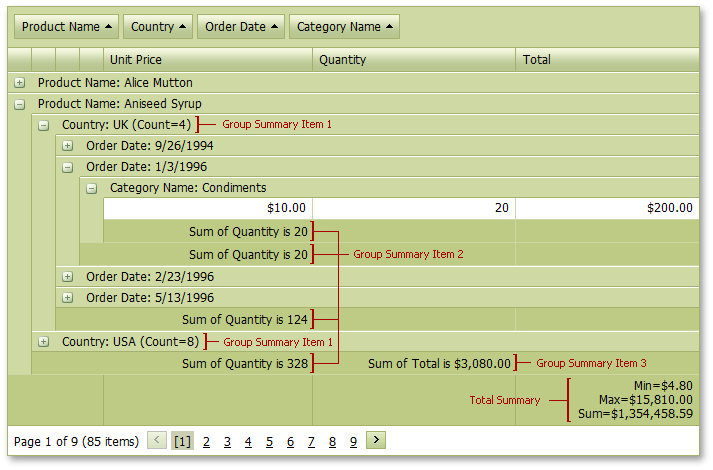20.2
20.1
19.2
19.1
18.2
18.1
17.2

# Use Data Summaries

ASPxGridView allows you to display a summary, i.e., brief information about groups of rows or individual data columns. For example, you can display the number of records or the maximum value.

ASPxGridView supports two types of summaries:

• Total Summary

A total summary displays the value of an aggregate function calculated across all rows within the ASPxGridView. The total summary is shown in the footer.

• Group Summary

A group summary displays the value of an aggregate function calculated across all rows within a group. The group summary is shown in the group row or group footer.• Custom Summary

A custom summary allows you to calculate summaries against records that meet specific criteria and use multiple data fields in calculations.

The ASPxGridView control maintains summaries as objects of the ASPxSummaryItem class. To work properly, the following two summary item properties must be specified.

``````<dx:ASPxSummaryItem FieldName="Total" SummaryType="Sum"/>
``````

The summary item above summarizes the 'Total' field values within a group and displays the sum in the group row.

The ASPxSummaryItem object contains the following two properties that allow you to specify the summary's display location.

##### NOTE

In server mode, a summary cannot be calculated for unbound columns in which events are used to calculate values (see ASPxGridView.CustomUnboundColumnData). Only columns with unbound expressions (see GridViewDataColumn.UnboundExpression) support summary calculation.

## Example

The code sample below demonstrates how you can specify group and total summaries. To specify the group summary items location, the ASPxSummaryItem.ShowInColumn and ASPxSummaryItem.ShowInGroupFooterColumn properties are used.

Note that summary item four is not displayed because the 'OrderDate' column, where the item should be displayed, does not exist (the grid is grouped by the 'OrderDate' field). Summary item five is not displayed because the 'Total' group row, where the item should be displayed, does not exist (the grid is not grouped by the 'Total' field)

The image below shows the result.``````<dx:ASPxGridView ID="grid" ...>
...
<TotalSummary>
<dx:ASPxSummaryItem FieldName="Total" SummaryType="Min" />
<dx:ASPxSummaryItem FieldName="Total" SummaryType="Max" />
<dx:ASPxSummaryItem FieldName="Total" SummaryType="Sum" />
</TotalSummary>

<GroupSummary>
<%-- Group Summary Item 1 --%>
<dx:ASPxSummaryItem FieldName="Country" ShowInColumn="Country" SummaryType="Count" />
<%-- Group Summary Item 2 --%>
<dx:ASPxSummaryItem FieldName="Quantity" ShowInGroupFooterColumn="UnitPrice" SummaryType="Sum" />
<%-- Group Summary Item 3 --%>
<dx:ASPxSummaryItem FieldName="Total" ShowInColumn="ProductName" ShowInGroupFooterColumn="Quantity" SummaryType="Sum" />
<%-- Group Summary Item 4 --%>
<dx:ASPxSummaryItem FieldName="Quantity" SummaryType="Sum" ShowInGroupFooterColumn="OrderDate" />
<%-- Group Summary Item 5 --%>
<dx:ASPxSummaryItem FieldName="Total" SummaryType="Sum" ShowInColumn="Total" />
</GroupSummary>
<Settings ShowGroupPanel="True" ShowFooter="True" ShowGroupFooter="VisibleIfExpanded"></Settings>
</dx:ASPxGridView>
``````